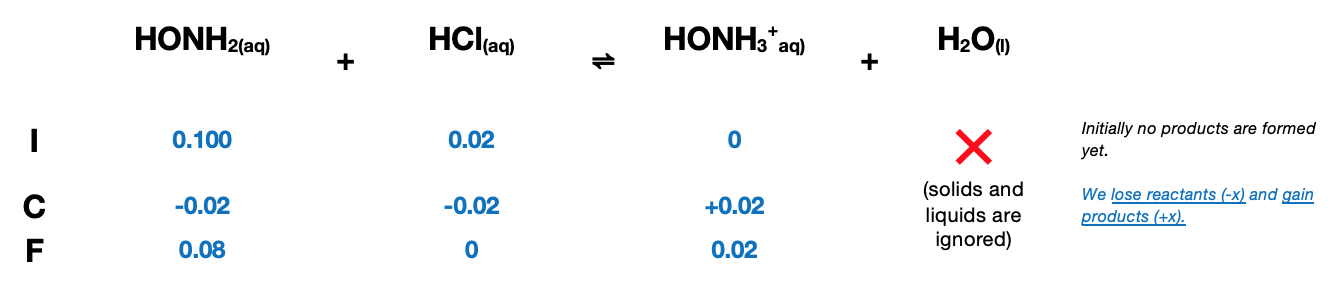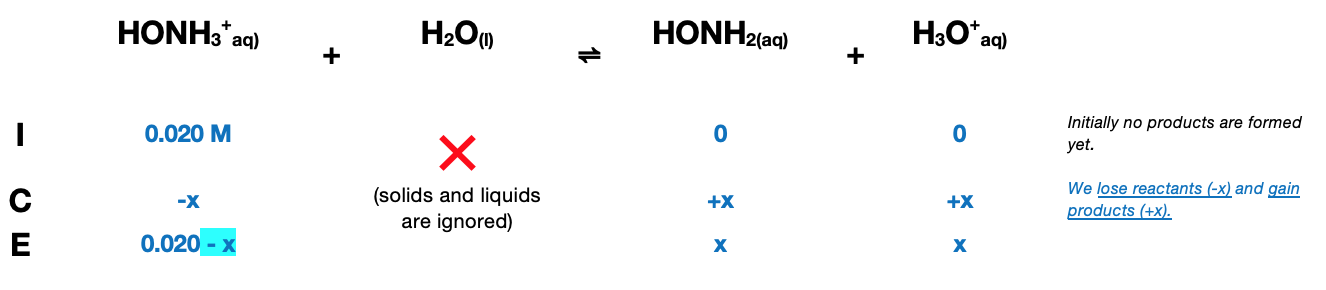# Problem: Calculate the pH after 0.020 mole of HCl is added to 1.00 L of each of the following solutions:a. 0.100 M HONH2 (Kb = 1.1 x 10-8)b. 0.100 M HONH3Clc. pure H2Od. a mixture containing 0.100 M HONH2 and 0.100 M HONH3Cl

###### FREE Expert Solution

For a.

0.100 mol

Reaction: HONH2(aq) + HCl(aq) ⇌ HONH3+(aq) + H2O(l)HONH3+(aq) + H2O(l) ⇌ HONH2(aq) + H3O+(aq)${\mathbf{K}}_{\mathbf{a}}\mathbf{=}\frac{{\mathbf{K}}_{\mathbf{w}}}{{\mathbf{K}}_{\mathbf{b}}}\mathbf{=}\frac{\mathbf{1}\mathbf{.}\mathbf{0}\mathbf{×}{\mathbf{10}}^{\mathbf{-}\mathbf{14}}}{\mathbf{1}\mathbf{.}\mathbf{1}\mathbf{×}{\mathbf{10}}^{\mathbf{-}\mathbf{8}}}\mathbf{=}{\mathbf{9}}{\mathbf{.}}{\mathbf{09}}{\mathbf{×}}{{\mathbf{10}}}^{\mathbf{-}\mathbf{7}}$

$\overline{){{\mathbf{K}}}_{{\mathbf{a}}}{\mathbf{=}}\frac{\mathbf{products}}{\mathbf{reactants}}{\mathbf{=}}\frac{\mathbf{\left[}{\mathbf{HONH}}_{\mathbf{2}}\mathbf{\right]}\mathbf{\left[}{\mathbf{H}}_{\mathbf{3}}{\mathbf{O}}^{\mathbf{+}}\mathbf{\right]}}{{{\mathbf{HONH}}_{\mathbf{3}}}^{\mathbf{+}}}}$

${{\mathbf{K}}}_{{\mathbf{a}}}{\mathbf{=}}\frac{\left(x\right)\left(x\right)}{\mathbf{0}\mathbf{.}\mathbf{020}\mathbf{-}\mathbf{x}}$

Solve for x:

${\mathbf{9}}{\mathbf{.}}{\mathbf{09}}{\mathbf{×}}{{\mathbf{10}}}^{\mathbf{-}\mathbf{7}}{\mathbf{=}}\frac{{x}^{2}}{\mathbf{0}\mathbf{.}\mathbf{020}\mathbf{-}\overline{)\mathbf{x}}}\phantom{\rule{0ex}{0ex}}\phantom{\rule{0ex}{0ex}}\sqrt{{\mathbf{x}}^{\mathbf{2}}}{\mathbf{=}}\sqrt{\left(9.09×{10}^{-7}\right)\left(0.020\right)}$

97% (479 ratings)###### Problem Details

Calculate the pH after 0.020 mole of HCl is added to 1.00 L of each of the following solutions:

a. 0.100 M HONH2 (Kb = 1.1 x 10-8)
b. 0.100 M HONH3Cl
c. pure H2O
d. a mixture containing 0.100 M HONH2 and 0.100 M HONH3Cl

Frequently Asked Questions

What scientific concept do you need to know in order to solve this problem?

Our tutors have indicated that to solve this problem you will need to apply the Buffer concept. You can view video lessons to learn Buffer. Or if you need more Buffer practice, you can also practice Buffer practice problems.

What professor is this problem relevant for?

Based on our data, we think this problem is relevant for Professor Rocher's class at OU.

What textbook is this problem found in?

Our data indicates that this problem or a close variation was asked in Chemistry: An Atoms First Approach - Zumdahl Atoms 1st 2nd Edition. You can also practice Chemistry: An Atoms First Approach - Zumdahl Atoms 1st 2nd Edition practice problems.